# Conics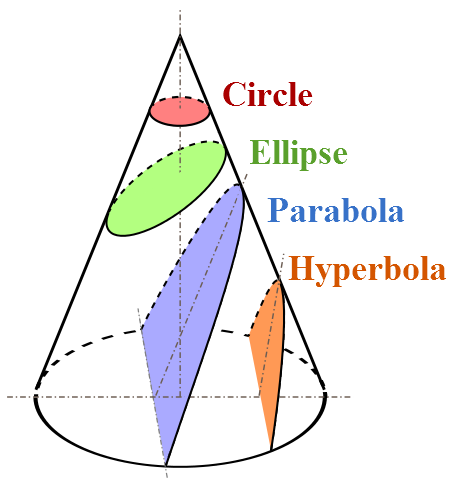There are 4 graphs that are formed when a cone is sliced by a flat surface. These conic sections are:

• Parabola
• Circle
• Ellipse
• Hyperbola

## Circle EquationsThe Extreme Orbiter above consists of five rotating arms with three pairs of spinning seats on each arm. The engineer who designed this carnival ride used this equation of a circle which is based on Pythagoras' Rule.

( x – h )2 + ( y – k )2 = r2

where
(h,k) is the centre
r is the radius of the circle.

## Example One

Find the coordinates of the centre and the length of the radius of a circle whose equation is:

( x – 3)2 + ( y + 4 )2 = 25.

Then draw this circle on grid paper.

Centre = (h,k) = (3, –4)## Ellipse Equations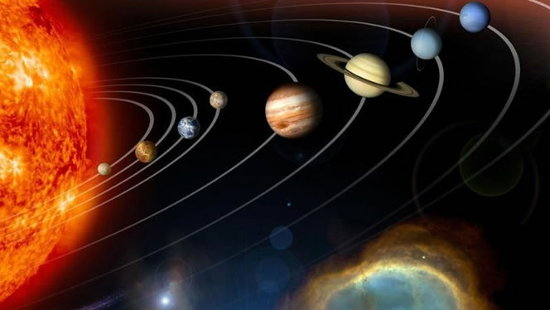( x – h )2 + ( y – k )2 = 1 a2 b2

where
(h,k) is the centre
a is half of the major axis
b is half of the minor axis of the ellipse.

## Example Two

Find the coordinates of the centre and the lengths of the axes whose equation is:

 ( x – 3 )2 + ( y + 4 )2 = 1 49 25

h = 3
k = –4
a = 7
b = 5

Centre = (h,k) = (3, –4)
Length of major axis = 7 × 2 = 14
Length of minor axis = 5 × 2 = 10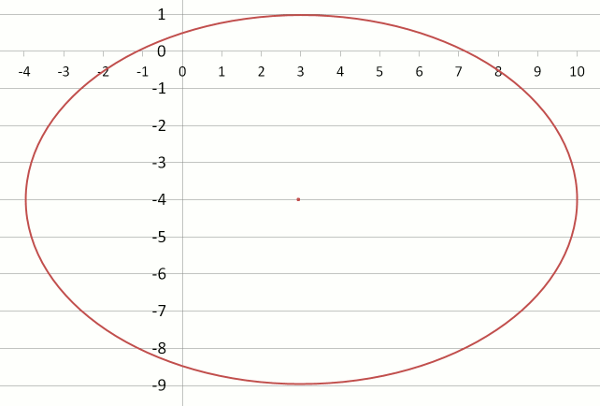## Hyperbola Equations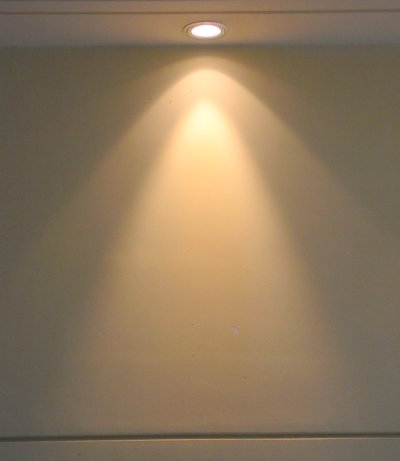The light given out by this lamp forms hyperbolas.

## Example Three

Draw the graphs of the following hyperbolic equations. What do you notice?

 (a) y = 1 x
 (b) y = 1 x – 3
 (c) y = 5 x – 3
 (d) y = 5 + 4 x – 3

(a)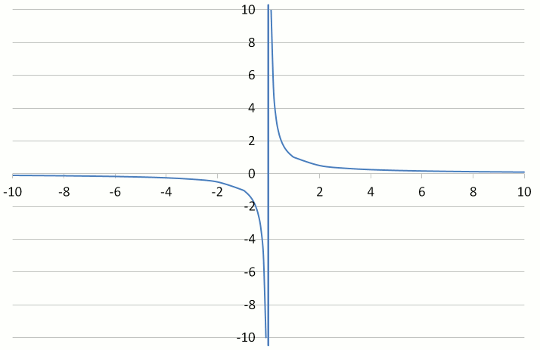(b)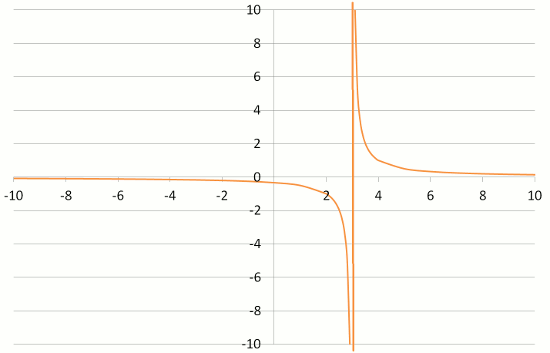(c)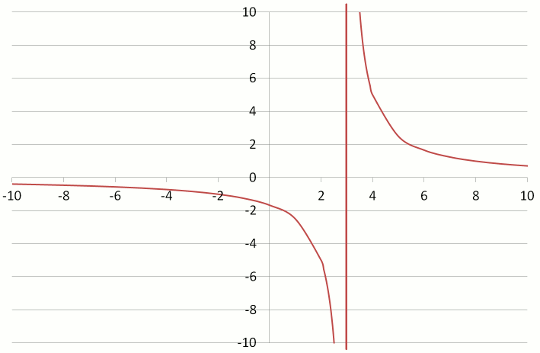(d)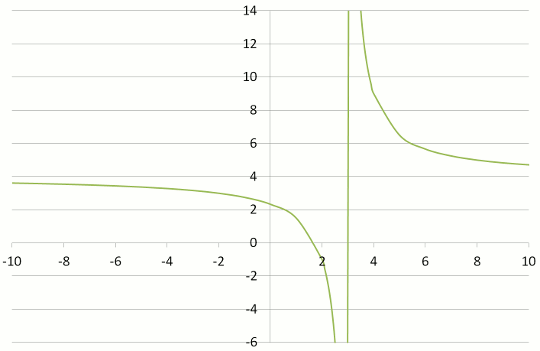## Maths Fun - Pizza PiecesBelow are the maximum numbers of pieces that you can get when making straight cuts of pizza.

• 0 cuts - 1 piece
• 1 cut - 2 pieces
• 2 cuts - 4 pieces
• 3 cuts - 7 pieces

By drawing a circle or more enjoyably, cutting (and then eating) a pizza, continue cutting and counting. If x is the number of cuts and y is the number of pieces, what is the equation that links these?